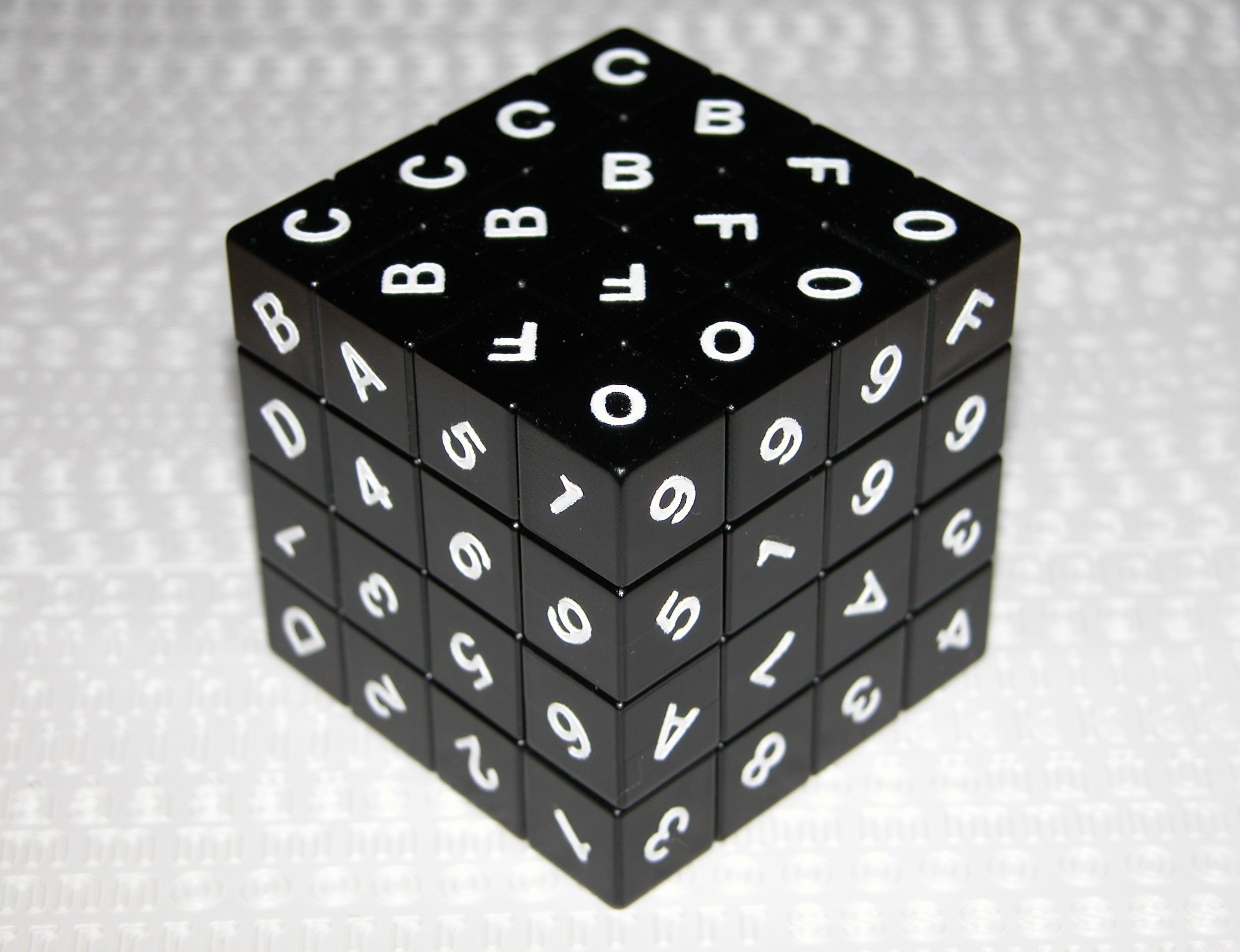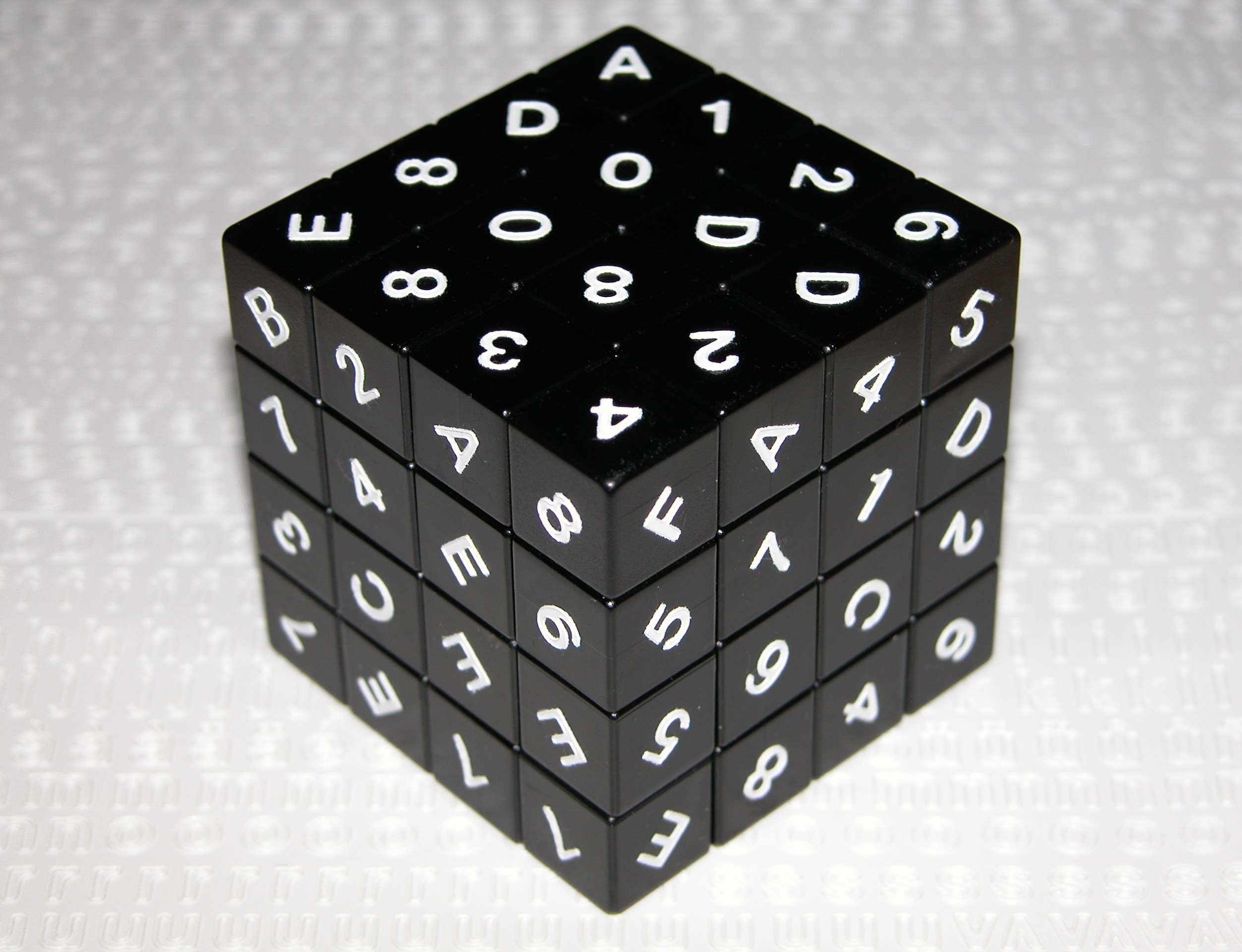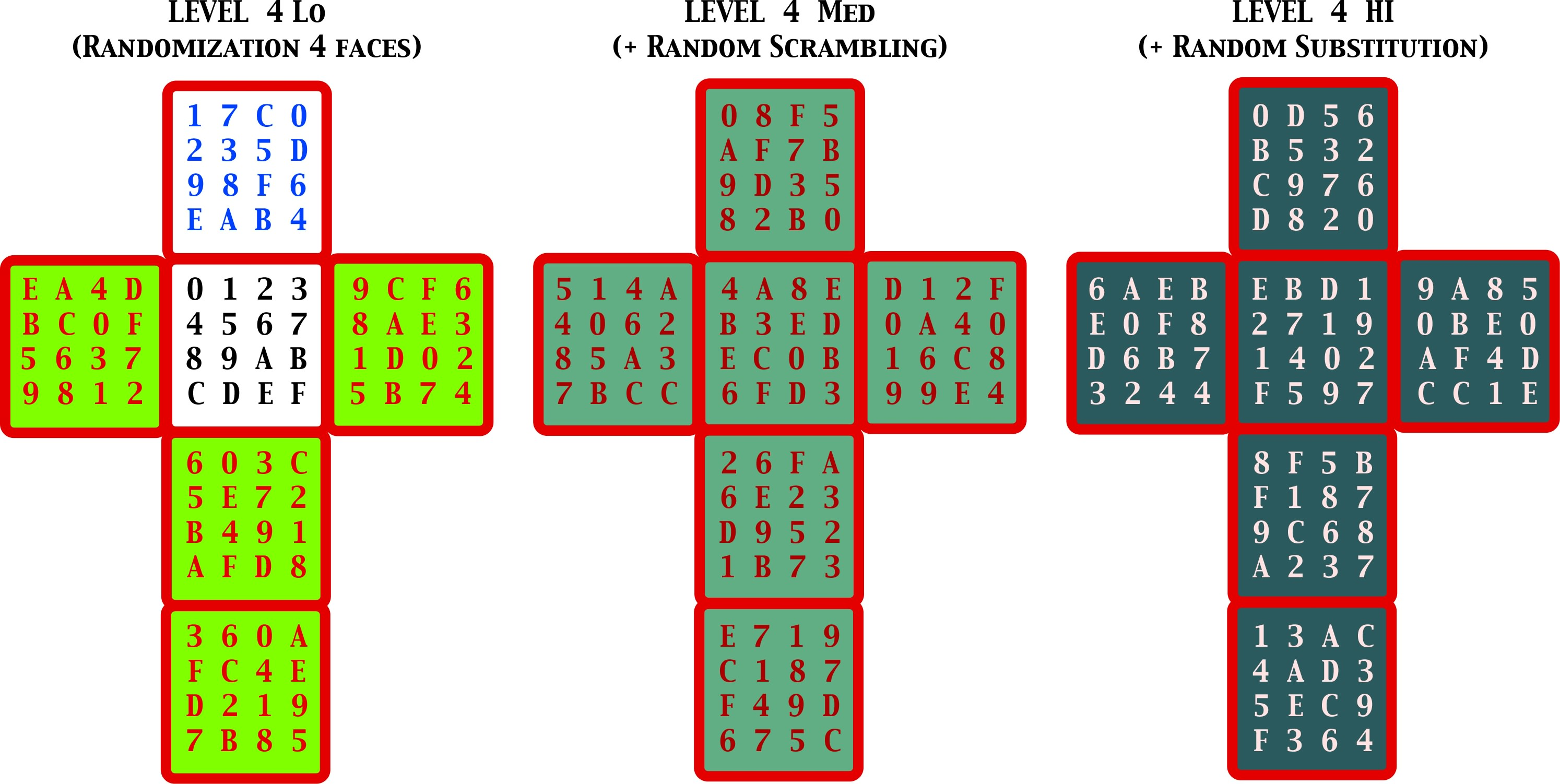I have constructed the following puzzle based on a 4x4x4 Eastsheen cube. It is labelled with hexadecimal digits 0,1,2,...,9,A,B,C,D,E,F on each face of each cubbie (I have turned the labels at an angle of 45 degrees to avoid having positional information due to label orientations). The goal of the puzzle is to have each label eaxctly once per face and once per row (in all 3 dimensions). This is the only constraint that must be satisfied. It seems possible that a single puzzle has more than one valid solved configuration, but I have not fund more than one so far. I wrote a computer program which generates the instance to be solved "randomly" and outputs the labelling of a scrambled puzzle. This last step is necessary to avoid knowing the solved configuration which would reduce the puzzle to returning each cubbie to its correct place... The puzzle is really difficult because the solved configuration is not known !!!an instance of the SudoKube Puzzle

The particular puzzle I am working with was generated with enough side information to return it to the solved configuration if I wish to look at the details of its generation.the 3 steps of generation of a random puzzle.

It was first generated by fixing a whole face to be the standard sequence 0,1,2,...,F. Then 4 faces (green) where generated randomly but so to satisfy the puzzle constraint (each digit once per face and once per row). The 6th face is uniquely determined by the first 5. The second step of generation was to scramble the cube with 200 random rotations. Finally the third step is to get rid of the fact that a fixed face was used in step one: a random permutation of the labels 0,1,2,...,F is generated and the labels are substituted in all 6 occurrences of each digit according to that permutation. I labelled my cube with the rightmost configuration and have been playing with it since then.

I am currently looking for an algorithm to solve my puzzle.

What I have been working with so far is to start by solving the corners, i.e. put them in a configuration where no label appears on two corners of a face or two corners of a row. There are roughly 3.5 million ways of arranging the corners. Using a computer program I discovered that a significant fraction (~1/300) of these satisfy the property. I have not yet completed any such valid set of corners further on to a full solution. My plan is to extend my program to first establish the set of all edges available and second the set of constraints on the edges once the corners are correctly arranged. I will then try to fit the edges to satisfy the constraints in a straight forward (greedy) manner.

Any idea is welcome...